## Saturday, February 28, 2015

### R.I.P. Leonard Nimoy

(Click on the comic if you can't see the full image.)He lived long. He prospered. Can us doing the same be part of his legacy?

It's been a while since I've included an obituary comic. I did a few very early in the run of (x, why?), but I had to stop. So many childhood idols were dying off, I could fill the strip with them ... except that I couldn't because my skills as an artist just weren't up to where they needed to be for a tribute. That's the main reason Gary Gygax didn't get a memorium strip (and that really bothered me at the time). As it is, if I hadn't taken the time not that long ago to create most of the artwork in today's comic, this might not have happened. Or it would've just been a picture of Leonard Nimoy and a quote with very little personal to it.

Whether or not to insert the character that sometimes is/sometimes isn't my own personal avatar was an easy question to answer. If I wanted to convey any personal emotion, it was the only way to boldly express it. Something I didn't do for so many of the other greats who have passed in the last 8 years that this strip has run -- people who inspired me or touched my life in some special way.

I never saw Leonard Nimoy in person. I was at one convention where I saw Mark Leonard and Jane Wyatt, (a.k.a. Spock's parents) and Nimoy was supposed to be there later in the evening, but we didn't stay that late. (It didn't help when we found out that they were only signing autographs on Sunday and we only had Saturday tickets). Another opportunity never came along. I envy the people who worked with him in his later years as he made guest appearances.

One thing I can take away from all this: from all the quotes that have been posted in social media, it's clear that Star Trek II: The Wrath of Khan is still the most memorable and meaningful of the Star Trek films (even if you prefer The Voyage Home). I've read the quotes, watched the funeral scene, heard the bagpipes.

I'll close with a different franchise. Some of you out there might not be Star Trek fans, and that's okay. But some of you might remember Nimoy from Mission: Impossible. Well, thanks to the magic of cable television and vintage programming, even though you won't get Spock, you'll always have Paris.## Wednesday, February 25, 2015

### (x, why?) Mini: Hyperbola

(Click on the comic if you can't see the full image.)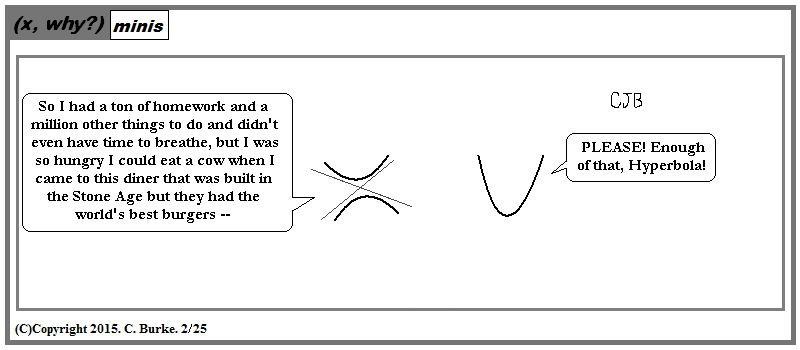Pronounced carefully, you can really put the bull in both ''hyperbole'' and ''hyperbola''.

Fun fact: you can sing "hyperbole, hyperbola!" in place of "funiculi, funicula!", but I don't know why you'd want to.## Monday, February 23, 2015

### Blanket Statement

(Click on the comic if you can't see the full image.)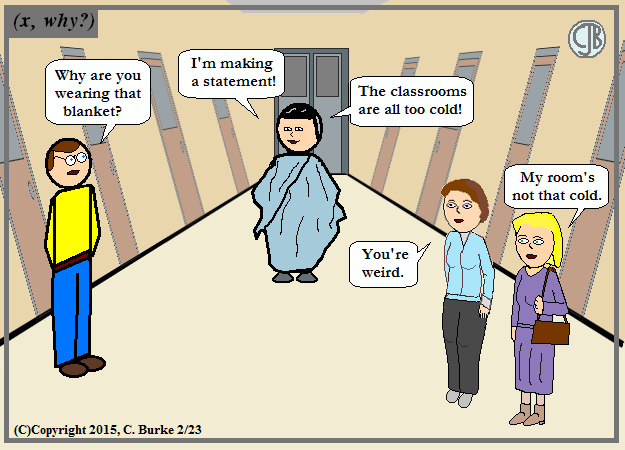Blanket statements are always wrong. Think about it.

I'm having a flashback to logic lessons where we took apart statements like "All the classrooms are too cold." and had to negate it properly. First thing, the opposite of "All" in NOT "None" (any more than "too cold" could be changed to "too warm"). The opposite of "All" is "Not All". Or "Some" (which could mean one because it would only take one room to disprove the statement). And then there was the ever-popular "There Exists a Room Such That ..."## Saturday, February 21, 2015

### (x, why?) Mini: Tessellate

(Click on the comic if you can't see the full image.)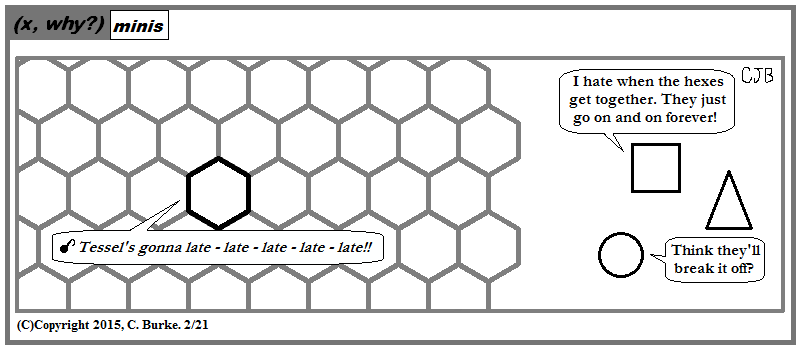Need a character name here. Tessel Six? Taylor Six-sided? I'll think about it.

Am I doing too much of the same stuff? Whatever, sue me. Unless you're part of the music industry -- then don't sue me -- I don't have any money.## Friday, February 20, 2015

### (x, why?) Mini: Diagonals

(Click on the comic if you can't see the full image.)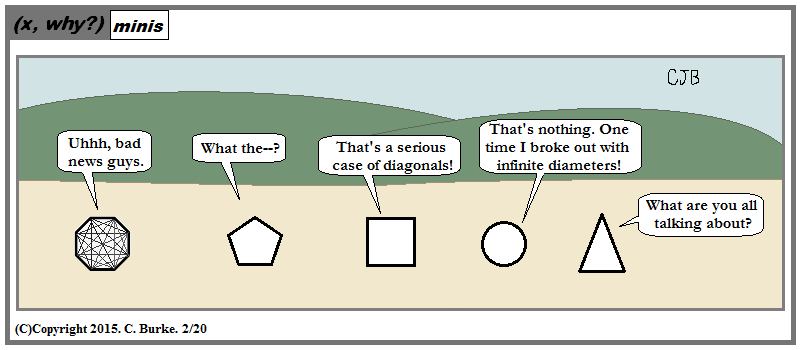Triangles have natural immunity to diagonals.

Playing with diagonals can be fun whether you're making Stars or Movies.## Thursday, February 19, 2015

### (x, why?) Mini: Cursive

(Click on the comic if you can't see the full image.)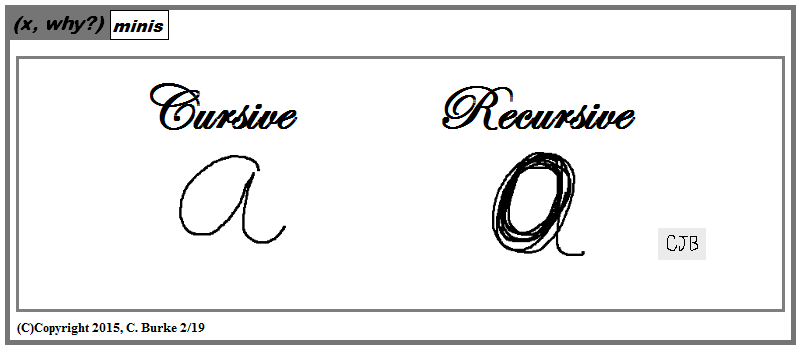Last week, a student mentioned he needed to learn cursive so he could sign autographs when he's a rap star. Except that (he says) all the good "P." rap names have been taken already.## Wednesday, February 18, 2015

### Outside the Box

(Click on the comic if you can't see the full image.)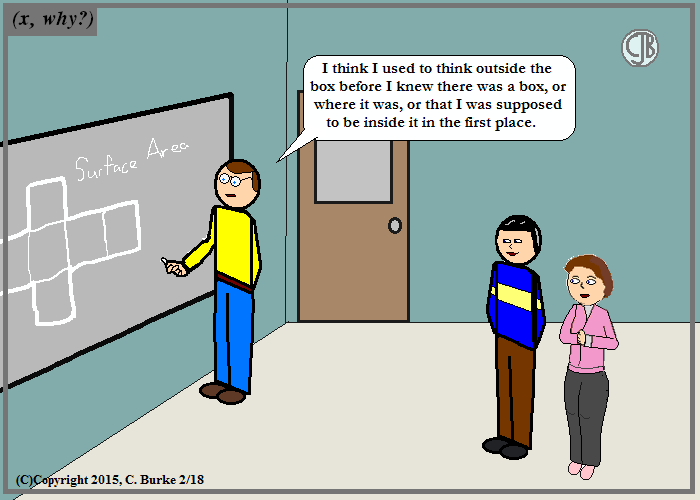I just went in and started teaching ... and then I was told how I was supposed to be doing it.

And when everyone is looking constantly over your shoulder, if your fresh way of thinking doesn't produce instant results, you fall into the trap of doing it someone else's way and, maybe, getting their results. Except that you were hired to do better than that.## Monday, February 16, 2015

I just got a heads-up that a recent Spiked Math comic, 573: Upper Heisenberg Matrix, links to my August 2010 comic Identity Matrix on the comics-only site, where no comments can be made. (I shut down the forum due to lack of use by anyone other than spammers and two dedicated followers ... and me.) Curious readers, as Spiked Math readers are known to be, just might click on the BLOG link and end up on the top post -- which for the moment is this one you're reading!

If you want to comment, the blog page for that comic is here.

Otherwise, if you're new, enjoy. Browse. Look at the pictures. Read the articles. (I know people who only read (x, why?) for the articles. Honest.) Have a good time. Tell your friends. Favorite, Like, +1, reddit whatever you, er, like. Link back to your favorites.

## Friday, February 13, 2015

### Five Things You Need to Know About Algebra, Part V

(Click on the comic if you can't see the full image.)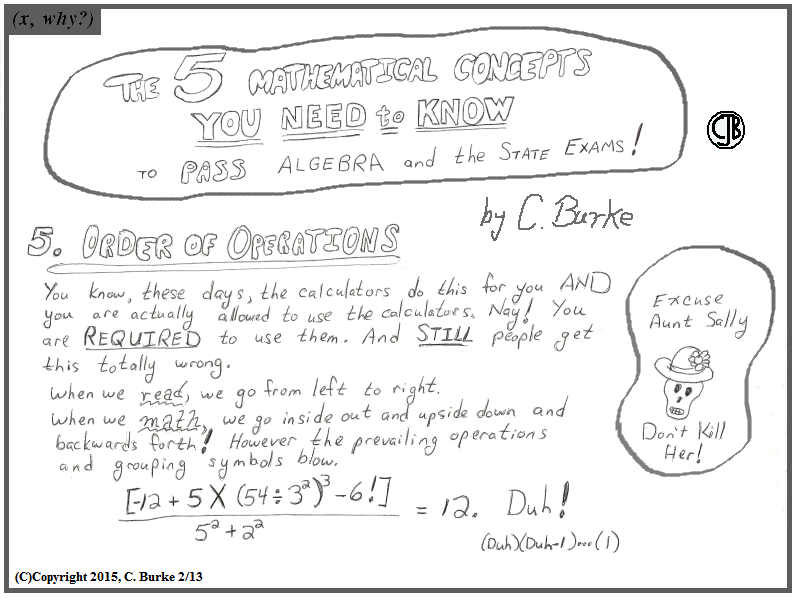I still can't believe I wanted to do this all in One Day as a Mega-comic.

As for the math: you'll notice that I actually left it out of the comic. I never heard "PEMDAS" until I became a teacher ... for a couple of years, no less. I learn Rules. Having a nice little code was nice at first, until I realized that students didn't understand the meaning behind the letters. Sure, they knew two things that they stood for, but the words didn't mean much to them. Worse, there was insistence that addition must come before subtraction, which isn't true.

Moreover, there are omissions. Where do the absolute brackets go? Well, there like Parentheses, but they aren't the same thing -- don't try any fancy Distributive Property stuff. And what about Factorial? Is it multiplication? No, it's more like exponentiation!

55 = 5 X 5 X 5 X 5 X 5
5! = 5 X 4 X 3 X 2 X 1

However, not the same thing because, for instance, 32 X 22 = 62 but (3!)(2!) =/= 6! -- and I'd like to add another exclamation point for emphasis!

By the way, I meant what I said about "two" things. I drew this Halloween comic (from 2007) on the board, and I student told me, "It's DEAR Aunt Sally! Don't you know anything?"

Could've been Aunt Silly for all that it mattered. And it was silly.## Thursday, February 12, 2015

### Five Things You Need to Know About Algebra, Part IV

(Click on the comic if you can't see the full image.)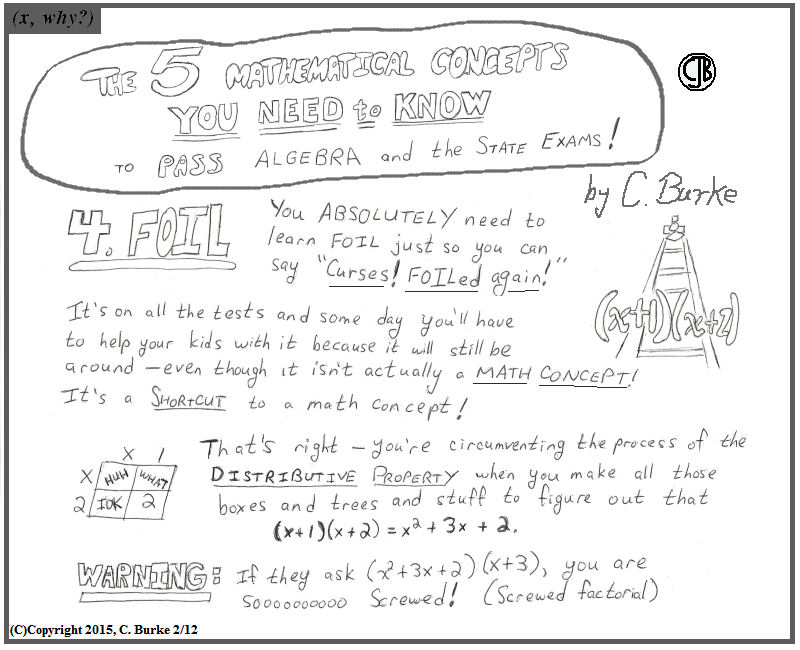I'd like to take an EPEE to FOIL. Or maybe a SABER.

Multiply everything inside the second set of parentheses by what's outside the parentheses, which in the case of two binomials is the first binomial. And then do it again with the other set of parentheses. This method will work with trinomials as well without leaving you scratching your head.

I've had students who could multiply a trinomial by a binomial because they didn't know what to do with the extra term because they'd learned a short-cut but not the concept.

Interestingly, when I try to demonstrate using integers instead of variables, the point gets lost because they finally start using the Order of Operations, correctly, and add what's inside the parentheses first. Sigh.## Wednesday, February 11, 2015

### Five Things You Need to Know About Algebra, Part III

(Click on the comic if you can't see the full image.)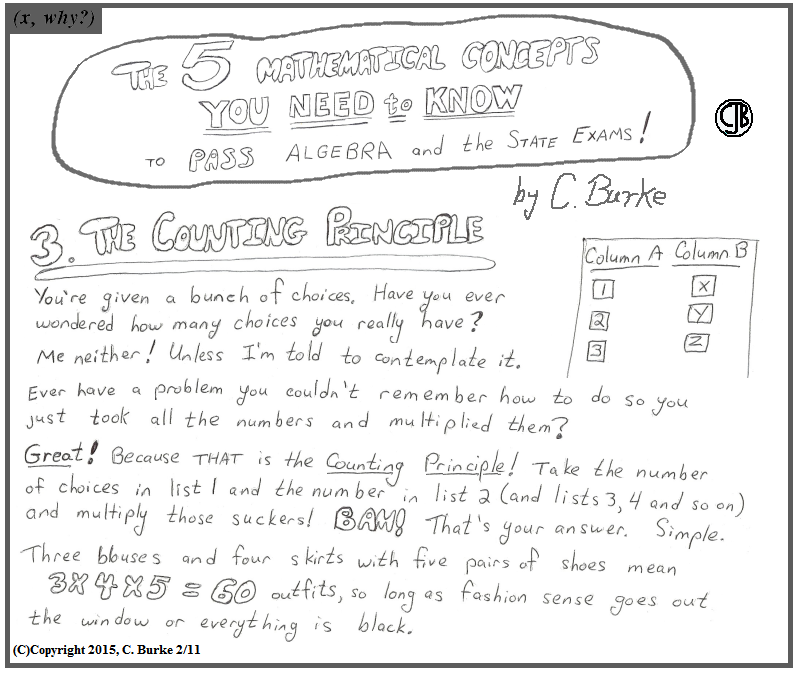And, yes, you are allowed to wear the same blouse two days in a row. With the same skirt even -- just change the shoes.

The biggest obstacle with the clothing problem wasn't what goes with what but the fact that you can reuse articles of clothing. The answer wasn't "Three, but you still have a skirt and some shoes leftover."

Similarly, the school cafeteria doesn't run out of chicken nuggets after the first student orders lunch.

I do have an old video online, but I've updated it in the past few years, and I haven't found a home for that version yet. I'll probably give in and put in on youtube.## Tuesday, February 10, 2015

### Five Things You Need to Know About Algebra, Part II

(Click on the comic if you can't see the full image.)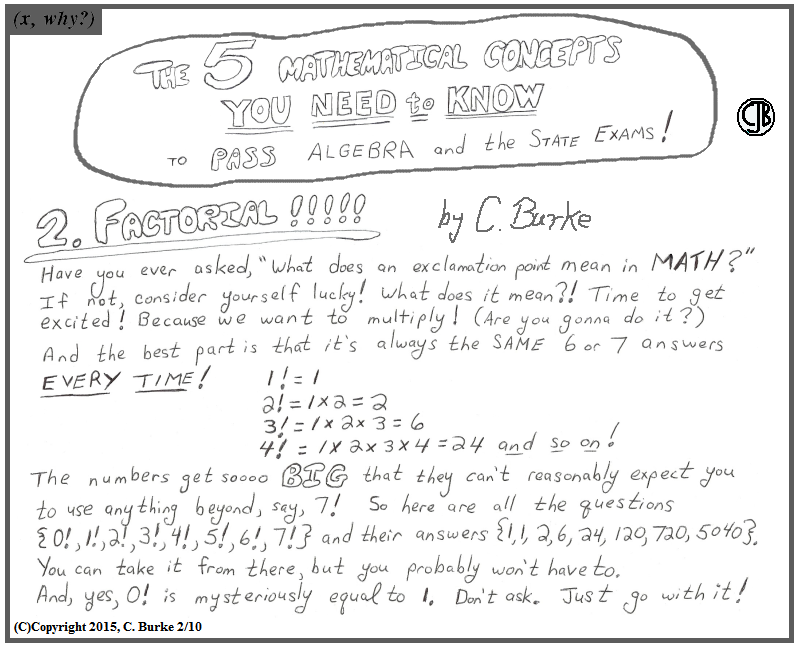True story: a student exiting the bathroom after a Regents exam, spotted me in the hall and asked, ''Mr. Burke, what does an exclamation point mean in a math problem?'' It didn't help that it was a meaningless probably and nothing to do with statistics or probability.

On another note: the number 5040 is very interesting. Not only is it 7 X 6 X 5 X 4 X 3 X 2 X 1, but it is also 10 X 9 X 8 X 7 (and that also means that 10!/7! = 6!). But here's another interesting fact: If the Moon was sitting on Earth's equator, the distance from the center of the Moon to the center of the Earth would be 5,040 miles. Unfortunately, if the Moon were sitting on Earth equator, it would be very bad because we would all die. Food for thought.## Monday, February 09, 2015

### Five Things You Need to Know About Algebra, Part I

(Click on the comic if you can't see the full image.)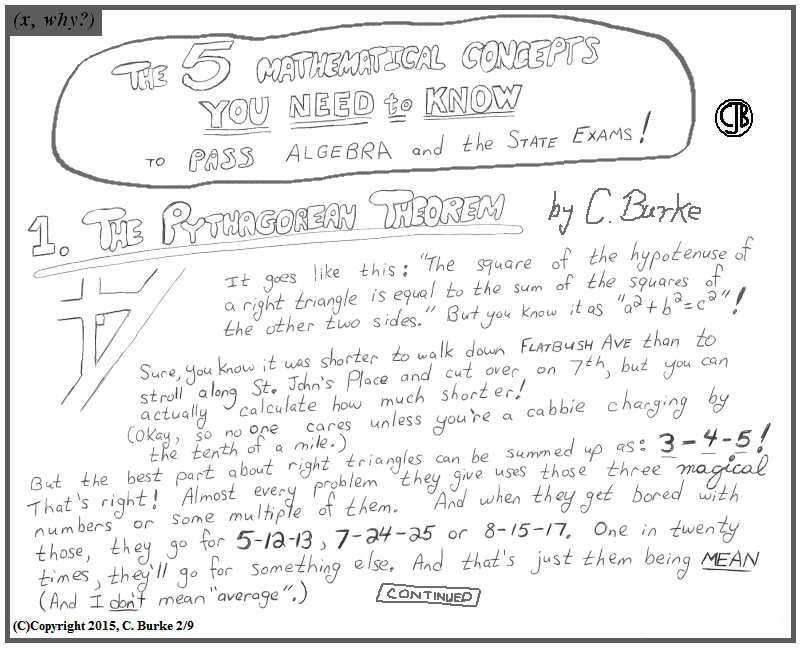Unless they want the third side rounded to the nearest tenth or expressed in simplest radical terms -- then you're on your own!

By the way, every multiple of the 3-4-5 right triangle has the same exact shape. They are similar figures so there angles are the same. And those angles will be 53 degrees (across from the "4" side) and 37 degrees (across from the "3" side). But show your Trigonometry work anyway like you didn't already know the answer in advance.## Thursday, February 05, 2015

### Speaking of Polygons

Today's comic had its genesis in a class a couple weeks back on finding the Measure of Interior Angles of Regular Polygons. As I have done in the past, the instructor (facilitator) informed the students that they wouldn't have to memorize the names of the polygons to answer questions, such as "Which polygon has seven sides?", but they would have to recognize the name of the polygon when they saw it and know how many sides it contained.

The majority of problems of this nature tend toward 5, 6, 8, 10 and 12-sided figures. (That's pentagon, hexagon, octagon, decagon and dodecagon for those of you playing along at home.) This could be because those are the easiest numbers to work with when it comes to the number 360 (yes, and 9, too, but...), and after five-sided pentagon, it's easier to draw regular shapes with even-numbered sides. (At least, that's my experience.)

Heptagon and nonagon were given, not just for the sake of completeness, but because they do turn up, even if not as often. After asking about how many they had to learn, one student asked "How many names are there?" A reasonable question. There are classifications for larger polygons, but they can default to n-gon, where n is the number of sides. And n can be 10 or 11 or 12, if we wanted it to be, but would more likely be 15 or 18 or 20, a larger number but one that would be easier to work with.

This had me wondering about the prefixes themselves. We had names for them up to 12, which corresponds to English words for numbers. We have a base 10, but we have names going up to 12 (which makes sense given imperial units). At thirteen, we start with "three and ten", then "four and ten", etc. However, in high school Spanish I, we had to learn names up to 15 -- once, doce, trece, catorce and quince -- (Yes, I remembered them, but I did double-check the spellings.) -- before we get to "ten and six", "ten and seven". So it's an arbitrary designation.

Years ago, at the request of a different student (obviously, as it was years ago), I looked up more names and found that an eleven-sided polygon was an undecagon. This made sense to me, as it was "one and ten", the way that dodecagon was "two and ten". And the pattern continued after that.

So when a student asked this class's instructor, "What about 11 sides?", I was a little surprised when she said, "A girl in my other class just looked that up. It's a hendecagon."

What?

Yes, I not-so-immediately, slightly nonchalantly, started typing on a computer in the corner. You learn new things. What did I learn?

Eleven in Greek is hendeka but in Latin it's undecim. The form undecagon is, therefore, a hybrid construction while the "cleaner" hendecagon is not.

This does lead to a problem with thirteen. The hybrid 13-sided name is tridecagon while the all-Greek variation would be triskaidecagon, which, while familiar to anyone who has heard of triskaidekaphobia, is nowhere nearly as easy to spell.

### (x, why?) Mini: Hendecagon

(Click on the comic if you can't see the full image.)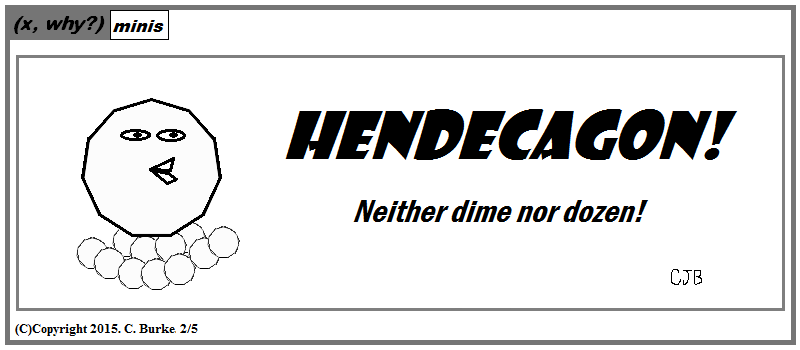Somewhere between metric and imperial lies Hendecagon.

UPDATE: Speaking of Polygons...## Wednesday, February 04, 2015

### January 2015 Integrated Algebra Regents exam, Multiple-Choice

Apologies for the delay: snow, a new job assignment, more snow, and the craziest with the testing. If anyone's still interested, here are the answers for the multiple-choice. Images will be added later. I still have to scan in some work for Part IV.

1. If A = {1, 2, 3, 4, 5, 6, 7, 8} and B = {2, 4, 6, 8, 10, 12}, the intersection of sets A and B is …

The intersection is comprised of the elements which appear in both sets. In this case {2, 4, 6, 8}.

2. What is the value of n in the equation 0.2(n – 6) = 2.8?

Work it out algebraically or work backward from the choices. It’s 20. You could either use the Distributive Property, or just divide both sides by 0.2.

3. The expression (24x6y3)/(-6x3y) is equivalent to …

Divide the coefficients and subtract the exponents: -4x3y2.

4. Which situation is represented by bivariate data?

“Bivariate” refers to two variables. The ice cream measuring temperature and sales is measuring two variables.

5. A cylinder has a circular base with a radius of 3 units and a height of 7 units. What is the volume of the cylinder in cubic units?

V = (pi)r2h = (pi)(3)2(7) = 63pi.

6. The graph of f(x) is shown below.

Based on this graph, what are the roots of the equation for f(x) = 0?

For what values of x is the function equal to 0? What are the roots of the function? Where does it cross the x-axis? At -1 and 5.

7. Jose wants to ride his bike a total of 50 miles this weekend. If he rides m miles on Saturday, which expression represents the number of miles he must ride on Sunday?

He rides whatever is left. Subtract 50 – m.

8. Four students are playing a math game at home. One of the math game questions asked them to write an algebraic equation.

 Brandon wrote 3(5x – 0) William wrote 7 < 2(6 + x) Alice wrote 15x Kayla wrote 11 = 2x + 3

Which student wrote an algebraic equation?

I commend these students for playing math games at home, but maybe they need to review some more if three of them got such a simple question incorrect! An equation has an equal sign (and not an inequality). Kayla wrote an equation.

9. A student spent 15 minutes painting a 2-foot by 3-foot bulletin board. To the nearest tenth of a minute, how long did it take the student to paint 1 square foot?

A 2’X3’ bulletin board is 6 square feet. Painting one-sixth of it would take 1/6th of 15, or 15/6 = 2.5

10. What is an equation of the line that passes through the points (2, 1) and (6, -5)?

If you use trial and error, you must check both points in the equation. Otherwise, find the slope: change in y over change in x is -6/4, which simplifies to -3/2. (You’ve eliminated 2 choices.) Now try (2, 1) in the equation – it doesn’t work in choice (1), but works in choice (2).

Yes, you could have plugged (2, 1) and -3/2 into y = mx + b, and solved for b as well.

11. What is 10/7x – 3/5x expressed in simplest form?

The least common denominator between 7x and 5x is 35x. Multiply the first fraction by (5/5) and the second by (7/7) to get 50/35x – 21/35x = 29/35x, which cannot be simplified.

12. In the box-and-whisker plot below, what is the 2nd quartile?

The 2nd quartile is also the median, which is the middle line on the plot, right above 30 on the number line.

13. Then length of a rectangle is three feet less than twice its width. If x represents the width of the rectangle, in feet, which inequality represents the area of the rectangle that is at most 30 square feet?

Area is length times width, which has to be less than or equal to 30: x(2x – 3) < 30.

14. Which set is a function?

The one where the x coordinate is not repeated is a function. In this case, choice (2).

15. The weights of 40 students were recorded. If the 75th percentile of their weights is 140 pounds, what is the total number of student s who weighed more than 140 pounds?

Three-quarters (75%) weight 140 or less. One-quarter (25%) of the 40 students weighed more. 25% of 40 is 10.

16. What is the slope of the line represented by the equation 4x + 3y = 7?

Subtract 4x from both sides. Divide the equation by 3. The slope is -4/3.
Also, if you are familiar with Standard Form of a linear equation (Ax + Bx = C), then you know that slope is –A/B.

17. What is sqrt(150) + sqrt(24)expressed in simplest radical form?

150 = 25 X 6 and 24 = 4 X 6. Sqrt(150) + sqrt(24) = 5*sqrt(6) + 2*sqrt(6) = 7*sqrt(6).

18. In triangle ABC, the measure of <A = 90 degrees, AB = 6, AC = 8 and BC = 10.

Which ratio represents the sine of <B?

Sine is opposite/hypotenuse or 8/10.

19. The equations 6x + 5y = 300 and 3x + 7y = 285 represent the money collected from selling gift baskets in a school fundraising event. If x represents the cost for each snack gift basket and y represents the cost for each chocolate gift basket, what is the cost for each chocolate gift basket?

Double the second equation and you get 6x + 14y = 570. Subtract the first equation and you get 9y = 270, so y = 30. The cost of a chocolate gift basket (y) is \$30.

20. Which equation represents the axis of symmetry of the graph of the equation y = x2 + 4x – 5?

When y = ax2 + bx + c, the axis of symmetry is x = -b/(2a), which means x = -4/2(1) = -2. So x = -2 is the equation.

21. For which value of x is the expression (x + 2)/(2x – 1) undefined?

We only care about the denominator and when it’s equal to zero.

2x – 1= 0
2x = 1
X = ½

22. Last year, Nick rode his bicycle a total of 8000 miles. To the nearest yard, Nick rode an average of how many yards per day? 1 mile = 1760 yards. 1 year = 365 days.

The conversion expression is
8000 miles/1 year * 1760 yards/ 1 mile * 1 year /365 days

The answer is 38575.342…., which rounds to 38,575.3

You didn’t actually have to do this much math! 8000 miles per year means that he’s going more than 20 miles per day (definitely more than 10 miles, right?) Look at the choices! Only one of them is greater than a single mile!

23. The set of integers is not closed for …

Division. If you add, subtract or multiply two integers, you will get another integer. If you divide two integers, more often than not, you will get a rational number that isn’t an integer.

24. A model rocket is launched into the air from ground level. The height, in feet, is modeled by p(x) = -16x2 + 32x, where x is the number of elapsed seconds. What is the total number of seconds the model rocket will be in the air.

Graph it. The roots will be 0 and 2. Those are the points where it’s on the ground.

25. The diagram below shows the path a bird flies from the top of a 9.5-foot-tall sunflower to a point on the ground 5 feet from the base of the sunflower.

To the nearest tenth of a degree, what is the measure of angle x?

You have the opposite and the adjacent, so the problem involves tangent. More specifically, tan-1.

Tan x = 5/.95
X = tan-1(5/95) = 79.24
Which to the nearest tenth is 79.2 degrees.

Tan x = 5/9.5
X = tan-1(5/9.5) = 27.76
Which to the nearest tenth is 27.8 degrees.

26. Which set of numbers represents the lengths of the sides of a right triangle?

You could use Pythagorean Theorem on this, or you could just recognize that 7, 24, 25 is a right triangle! 49 + 576 = 625.

27. How many different seven-letter arrangements of the letters in the word HEXAGON can be made if each letter is used only once?

7!, which is 7 X 6 X 5 X 4 X 3 X 2 X 1 or 5040.

28. Three students each rolled a wooden cube with faces painted red, white and blue. The color of the top face is recorded each time the cube is rolled. The table below shows the results.

If a fourth student rolled the cube 75 times, based on these experimental data, approximately how many times can the cube be expected to land with blue on top?

In all three of the cases of the other students, blue came out exactly 40% (2/5ths) of the time. Note: Experimental data never turns out like this. Nonetheless, you are expected to answer 40% of 75, which is 29.6. Since the answer has to be a whole number, round up to 30.

You might also have noted that the sum of students 2 and 3 have blue occurring 28 out of 70 times. Five more rolls can’t get it to 35 or 40, so 30 is the only choice which makes sense.

29. Dominick graphs the equation y = a|x| where a is a positive integer. If Gina multiplies a by -3, the new graph will become …

Narrower (because of 3) and open downward (because of the negative sign).

30. Mr. Suppe recorded the height, in inches, of each student in his class. The results are recorded in the table below.

Which cumulative frequency histogram represents the data?

First, (1) and (2) are not cumulative, so they can be eliminated. Second, there are 20 pieces of data, but choice (3) only goes up to 10. There is no need to actually sort the data or make a table. The answer is obviously (4).

## Sunday, February 01, 2015

### XLIX

(Click on the comic if you can't see the full image.)